# Descriptive statistics for Individual Distribution Identification

Find definitions and interpretation guidance for every descriptive statistic that is provided with Individual Distribution Identification.

## N

The number of nonmissing values in the sample. N is the count of all the observed values.

In this example, there are 141 recorded observations.
Total N N*
149 141 8

### Interpretation

Use N to assess your sample size.

Generally, larger samples produce more reliable results for assessing the distribution fit.
###### Important

Use caution when you interpret results from a very small or a very large sample. If you have a very small sample, a goodness-of-fit test may not have enough power to detect significant deviations from the distribution. If you have a very large sample, the test may be so powerful that it detects even small deviations from the distribution that have no practical significance. Use the probability plots in addition to the p-values to evaluate the distribution fit.

## N*

The number of missing values in the sample. N* is the count of the cells in the worksheet that contain the missing value symbol *.

In this example, 8 errors occurred during data collection and are recorded as missing values.
Total N N*
149 141 8

## Mean

The mean is calculated as the average of the data, which is the sum of all the observations divided by the number of observations.

For example, the waiting time (in minutes) of five customers in a bank are: 3, 2, 4, 1, and 2. The mean waiting time is calculated as follows: On average, a customer waits 2.4 minutes for service at the bank.

### Interpretation

Use the mean to describe the sample with a single value that represents the center of the data. Many statistical analyses use the mean as a standard reference point.

The median and the mean both measure central tendency. But unusual values, called outliers, generally affect the median less than they affect the mean. If your data are symmetric, the mean and median are similar.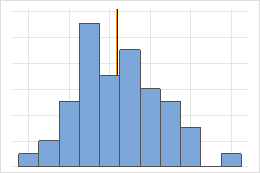For the symmetric distribution, the mean (blue line) and median (orange line) are nearly the same. Therefore, the lines overlap and cannot be distinguished from one another. For the non-symmetric distribution, the data is skewed to the right, which causes the mean value to be greater than the median.

## StDev

The standard deviation (StDev) is the most common measure of dispersion, or how spread out the data are about the mean. The symbol σ (sigma) is often used to represent the standard deviation of a population, and s is used to represent the standard deviation of a sample.

### Interpretation

Use the standard deviation to determine how spread out the data are from the mean. A larger sample standard deviation indicates that your data are spread more widely around the mean.

You can also use the standard deviation to establish a benchmark for estimating the overall variation of a process. Variation that is random or natural to a process is often called noise.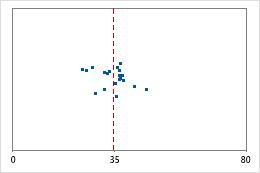Hospital discharge timesAdministrators track the discharge time for patients who are treated in the emergency departments of two hospitals. Although the average discharge times are about the same (35 minutes), the standard deviations are significantly different. The standard deviation for hospital 1 is about 6. On average, a patient's discharge time deviates from the mean (dashed line) by about 6 minutes. The standard deviation for hospital 2 is about 20. On average, a patient's discharge time deviates from the mean (dashed line) by about 20 minutes.

## Median

The median is the midpoint of the data set. This midpoint value is the point at which half of the observations are above the value and half of the observations are below the value. The median is determined by ranking the observations and finding the observation at the number [N + 1] / 2 in the ranked order. If the number of observations is even, the median is the value between the observations ranked at numbers N / 2 and [N / 2] + 1.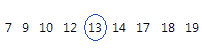For this ordered data, the median is 13. That is, half of the values are less than or equal to 13, and half of the values are greater than or equal to 13.

### Interpretation

The median and the mean both measure central tendency. But unusual values, called outliers, generally affect the median less than they affect the mean. If your data are symmetric, the mean and median are similar.For the symmetric distribution, the mean (blue line) and median (orange line) are nearly the same. Therefore, the lines overlap and cannot be distinguished from one another. For the non-symmetric distribution, the data is skewed to the right, which causes the mean value to be greater than the median.

## Minimum

The smallest data value.

In these data, the minimum is 7.

 13 17 18 19 12 10 7 9 14

### Interpretation

Use the minimum to identify a possible outlier. If the value is unusually low, investigate its possible causes, such as a data-entry error or a measurement error.

One of the simplest ways to assess the spread of the data is to compare the minimum and maximum to determine its range. The range is the difference between the maximum and the minimum value in the data set. When you evaluate the spread of the data, also consider other measures, such as the standard deviation.

## Maximum

The largest data value.

In these data, the maximum is 19.

 13 17 18 19 12 10 7 9 14

### Interpretation

Use the maximum to identify a possible outlier. If the value is unusually high, investigate its possible causes, such as a data-entry error or a measurement error.

One of the simplest ways to assess the spread of the data is to compare the minimum and maximum to determine its range. The range is the difference between the maximum and the minimum in the data set. When you evaluate the spread of the data, also consider other measures, such as the standard deviation.

## Skewness

Skewness is the extent to which the data are not symmetrical.

### Interpretation

Use skewness to obtain an initial understanding of the symmetry of your data.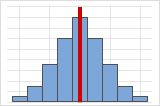Symmetrical or non-skewed distributionsAs data becomes more symmetrical, its skewness value approaches 0. Figure A shows normally distributed data, which by definition exhibits relatively little skewness. The line in middle of the histogram of normal data shows that the two sides mirror one another. Lack of skewness by itself, however, does not imply normality. Figure B shows a distribution where the two sides mirror one another, but the data is not normally distributed.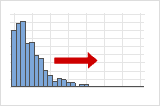Positive- or right-skewed distributionsPositive-skewed data is also called right-skewed data because the "tail" of the distribution points to the right. Positive-skewed data has a skewness value that is greater than 0. Salary data often is positively skewed: many employees in a company make relatively low salaries while increasingly few people make very high salaries.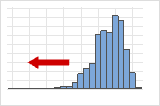Negative- or left-skewed distributionsNegative-skewed data is often called left-skewed data because the "tail" of the distribution points to the left. Negative-skewed data has a skewness value that is less than 0. Failure rate data is often negatively skewed. For example, very few light bulbs burn out immediately, and most bulbs do not burn out for a long time.

## Kurtosis

Kurtosis indicates how the tails of a distribution differ from the normal distribution.